Learning Library
Who we are
Guidance

# Addition Math Puzzles

These fun math puzzles are a great way for second graders to dig into math skills.

Click on a worksheet in the set below to see more info or download the PDF.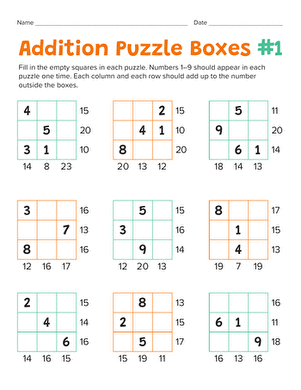### Addition Math Puzzles

Put your second grader's math skills to the test with mathematical puzzle boxes. He'll practice doing addition facts in his head, and build logic skills.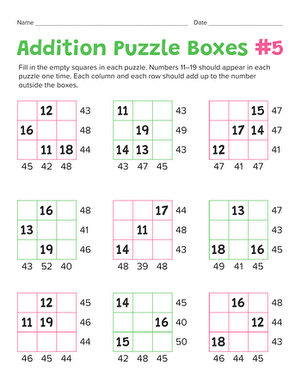### Addition Math Puzzles #2

Your second grader will practice adding up to 50 while building her logic and problem solving skills with these math puzzle boxes.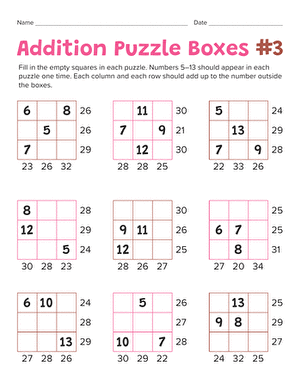### Addition Math Puzzles #3

Put your child's addition abilities to the test with mathematical puzzle boxes. He'll practice his addition as he solves each puzzle.### Addition Math Puzzles #4

Math lovers can put their addition and subtraction skills to the test to figure out these Sudoku-style puzzles, a perfect introduction to basic algebra!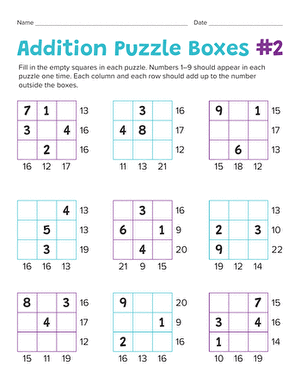### Addition Math Puzzles #5

Make practicing math fun with math puzzles. Your child will learn the basics of solving logic problems as he does math in his head.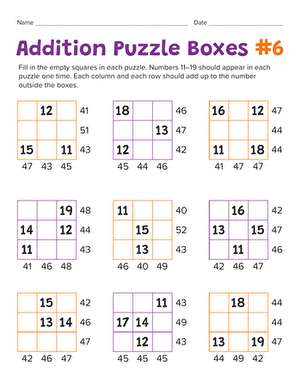### Addition Math Puzzles #6

Add a fun twist to math practice with these addition puzzles. Your math maniac will get an introduction to logic and problem solving.

The set is continued below.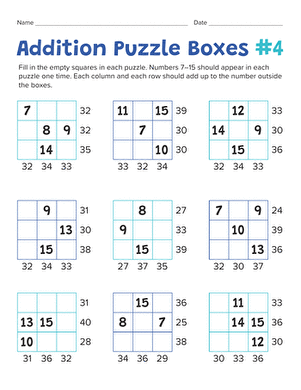### Addition Math Puzzles #7

What better way to learn math than with an addition puzzle? Your math star will practice addition and get a taste of problem solving with logic.